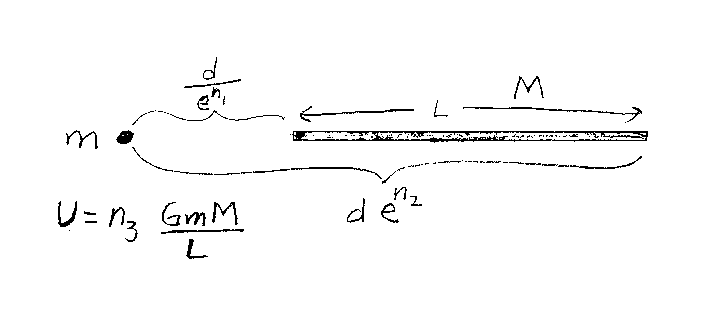Problem B8: Consider the two object below. One is very small (point object) with mass m, and the other is a very thin rod of length L, mass M, whose mass is uniformly distributed. The object of mass m is located a distance of d/en1 from the left end and a distance of den2 from the right end of the rod. What is the gravitational potential energy of the objects? If the gravitational energy is U=n3 GmM/L, what is n3? Note that all the integers are unitless, and that the reference U=0 is when they are infinitely far apart.n1 = n2 = Input n3:

If you are currently in my class, you can record your grade by entering your name and student ID number (without the leading zeros) below and clicking on "record grade".
 First Name = Last Name = ID = Problem: